# C++ Basic Operations on Binary Files

Let's now learn about basic operations on files. The operations that you have learnt so far are applicable on both text and binary files. The only difference is that while working with binary files, you do need to prefix file modes with ios::binary at the time of opening the file. You have learnt how to create files (open them in ios::out or ios::app mode which will create a file if it doesn't exist already), how to read records from binary files (call read() function through stream object to which file is attached), how to write records in binary files (call write() function through stream object which file is attached.).

Here, in this tutorial, we will perform the following basic operations on binary files:

• searching
• appending data
• inserting data in sorted file
• deleting a record
• modifying data

## Searching in C++

We can perform search in a binary file opened in input mode by reading each record then checking whether it is our desired record or not. For instance, if you want to search for a record for a student having rollno as 1 in file marks.dat, you can implement this search process in C++ in two manners :

1. with records implemented through structures.
2. with records implemented through classes.

When records are implemented through structures, you can perform search as demonstrated in the following examples :

```struct student
{
int rollno;
char name;
char branch;
float marks;
}stud1;

ifstream fin("marks.dat", ios::in | ios::binary);
:          		      // Read rollno to be searched for

while(!fin.eof())
{
if(stud1.rollno == rn)                  // if true, record is found
{
:                  // process desired record here

found = 'y';       // after processing you may jump from the
break;             // loop employed form searching purpose
}
}

{
:                          // display error message here or process as desired
}```

Let's take an example for complete understanding on searching operation in C++.

### C++ Searching Example

Here is an example program, demonstrating the searching operation on binary files in C++.

```/* C++ Basic Operations on Binary Files
* This program demonstrates the searching
* operation in a C++ program. Here the
* searching operations performed, on
* the records implemented through structures
*/

#include<fstream.h>
#include<conio.h>
#include<stdlib.h>

class student
{
int rollno;
char name;
char branch;
float marks;

public:
void getdata()
{
cout<<"Rollno: ";
cin>>rollno;
cout<<"Name: ";
cin>>name;
cout<<"Branch: ";
cin>>branch;
cout<<"Marks: ";
cin>>marks;

if(marks>=75)
{
}
else if(marks>=60)
{
}
else if(marks>=50)
{
}
else if(marks>=40)
{
}
else
{
}
}

void putdata()
{
cout<<"Rollno: "<<rollno<<"\tName: "<<name<<"\n";
}

int getrno()
{
return rollno;
}
}stud1;

void main()
{
clrscr();

fstream fio("marks.dat", ios::in | ios::out);
char ans='y';
while(ans=='y' || ans=='Y')
{
stud1.getdata();
fio.write((char *)&stud1, sizeof(stud1));
cout<<"\nWant to enter more ? (y/n)..";
cin>>ans;
}

clrscr();
int rno;
long pos;
char found='f';

cout<<"Enter rollno of student to be search for: ";
cin>>rno;

fio.seekg(0);
while(!fio.eof())
{
pos=fio.tellg();
if(stud1.getrno() == rno)
{
stud1.putdata();
fio.seekg(pos);
found='t';
break;
}
}
if(found=='f')
{
cout<<"Press any key to exit...\n";
getch();
exit(2);
}

fio.close();
getch();
}```

Here are the sample run of the above C++ program:After entering the three records, press n and then ENTER. Now to search for a specific roll number. Enter the roll number of a student and press enter to perform searching operation. Here is the sample run: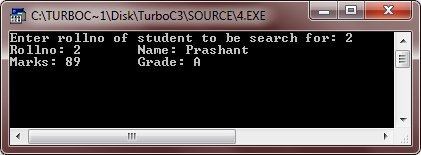When the records are implemented through classes, then you may need to add an additional accessor function in public: section of the class, that returns the value of the data member to which the user-specified search value is to be compared. With structures, this was not required as all the data members were public by default and hence accessible through structure variable. On the other hand, through an object, private data members can't be accessed. Therefore, to read value of a private data member, a function is added in the public section, that returns this value. The rest of the processing is just similar to that of the structures. Here is an example code fragment demonstrates this:

```class student
{
int rollno;
char name;
char branch;
float marks;

public:
void getdata();
void putdata();
int getrno()	 // this function returns the value of private member rollno
{
return rollno;
}
}stud1;

ifstream fin("marks.dat", ios::in);
:		// read rollno to be search for

while(!fin.eof())
{
if(stud1.getrno() == rno)		// retrieve value of private member and compare
{
:		// process desired record here

found = 't';
break;
}
}
{
}```

Let's take an example for the complete understanding on the searching operations on the records implemented through classes in C++.

```/* C++ Basic Operations on Binary Files
* This program demonstrates the searching
* operation in a C++ program. Here the
* searching operations performed, on
* the records implemented through classes
*/

#include<fstream.h>
#include<conio.h>
#include<stdlib.h>

class student
{
int rollno;
char name;
char branch;
float marks;

public:
void getdata()
{
cout<<"Rollno: ";
cin>>rollno;
cout<<"Name: ";
cin>>name;
cout<<"Branch: ";
cin>>branch;
cout<<"Marks: ";
cin>>marks;

if(marks>=75)
{
}
else if(marks>=60)
{
}
else if(marks>=50)
{
}
else if(marks>=40)
{
}
else
{
}
}

void putdata()
{
cout<<name<<", rollno "<<rollno<<" has ";
}

int getrno()
{
return rollno;
}
}stud1;

void main()
{
clrscr();

ofstream fout("marks.dat", ios::out);
char ans='y';
while(ans=='y' || ans=='Y')
{
stud1.getdata();
fout.write((char *)&stud1, sizeof(stud1));
cout<<"\nWant to enter more ? (y/n)..";
cin>>ans;
}
fout.close();

clrscr();
int rno;
char found;
ifstream fin("marks.dat", ios::in);

found = 'n';
cout<<"Enter rollno to be searched for: ";
cin>>rno;

while(!fin.eof())       // end-of-file used here
{
if(stud1.getrno() == rno)
{
cout<<"Record found at roll number "<<rno<<". Here is the record\n";

stud1.putdata();
found = 't';
break;
}
}
if(found=='n')
{
cout<<"Press any key to exit...\n";
getch();
exit(2);
}

fin.close();
cout<<"\nPress any key to exit...\n";
getch();
}```

Here are the sample runs of the above C++ program: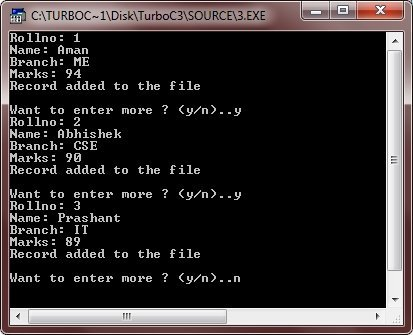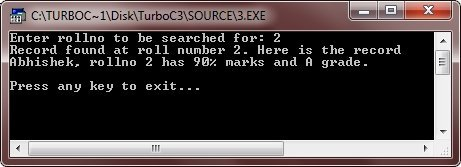## Appending Data in C++

To append data in a file, the file is opened with the following two specifications :

1. file is opened in output mode
2. file is opened in ios::app mode

Once the file gets opened in ios::app mode, the previous records/information is retained and new data gets appended to the file.

Let's see the following code fragment that appends new records to file marks.dat :

```class student
{
:
}stud1;
ofstream fout;
fout.open("marks.dat", ios::app } ios::binary);
/* Repeat following lines as many times */
{
fout.write((char *) & stud1, sizeof(stud1));    // record appended
}```

### C++ Appending Data Example

Here is an example program demonstrates, how to append data in a file in C++.

```/* C++ Basic Operations on Binary Files
* This program demonstrates, how to
* append data in a file in C++ */

#include<fstream.h>
#include<conio.h>
#include<stdlib.h>

class student
{
int rollno;
char name;
char branch;
float marks;

public:
void getdata()
{
cout<<"Rollno: ";
cin>>rollno;
cout<<"Name: ";
cin>>name;
cout<<"Branch: ";
cin>>branch;
cout<<"Marks: ";
cin>>marks;

if(marks>=75)
{
}
else if(marks>=60)
{
}
else if(marks>=50)
{
}
else if(marks>=40)
{
}
else
{
}
}

void putdata()
{
cout<<name<<", rollno "<<rollno<<" has ";
}

int getrno()
{
return rollno;
}
}stud1;

void main()
{
clrscr();

ofstream fout("marks.dat", ios::app);
char ans='y';
while(ans=='y' || ans=='Y')
{
stud1.getdata();
fout.write((char *)&stud1, sizeof(stud1));
cout<<"Data appended in the file successfully..!!\n";
cout<<"\nWant to enter more ? (y/n)..";
cin>>ans;
}

fout.close();
cout<<"\nPress any key to exit...\n";
getch();
}```

Here is the sample run of this C++ program: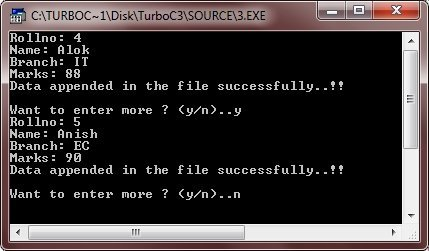## C++ Inserting Data in Sorted File

To insert data in a sorted file, firstly, its appropriated position is determined and then records in the file prior to this determined position are copied to temporary file, followed by the new record to be inserted and then the rest of the records from the file are also copied.

For example, records in marks.dat are sorted in ascending order on the basis of rollno. Assuming that there are about 10 records in the file marks.dat. Now a new record with rollno 5 is to be inserted. It will be accomplished as follows :

(i) Determining the appropriate position. If the rollno of new record say NREC in which rollno 5 is lesser than the rollno of very first record, then the position is 1, where the new record is to be inserted as it will be inserted in the beginning of the file to maintain the sorted order.

If the rollno of new record (5 here) satisfies following condition for two consecutive records say (pth and (p + 1)th)

`if prev.getrno() <= NREC.getrno() && (NREC.getrno() <= next.getrno())`

then the appropriate position will be position of prev + 1 i.e, p + 1.

And if the rollno of the new record is more than the rollno of last record (say nth record) then the appropriate position will be n+1.

(ii) Copy the records prior to determined position to a temporary file say temp.dat.

(iii) Append the new record in the temporary file temp.dat.

(iv) Now append the rest of the records in temporary file temp.dat.

(v) Delete the file marks.dat by using the following code.

`remove("marks.dat");`

(vi) Now, rename the file temp.dat as marks.dat as follows :

`rename("temp.dat", "marks.dat");`

Let's take an example for the complete understanding on inserting data in a sorted file in C++.

### C++ Inserting Data in Sorted File Example

Here is an example program demonstrating, how to insert data in a sorted file in C++

```/* C++ Basic Operations on Binary Files
* This program demonstrates how to insert
* data in a sorted file in C++ */

#include<fstream.h>
#include<conio.h>
#include<stdio.h>
#include<stdlib.h>

class student
{
int rollno;
char name;
char branch;
float marks;

public:
void getdata()
{
cout<<"Rollno: ";
cin>>rollno;
cout<<"Name: ";
cin>>name;
cout<<"Branch: ";
cin>>branch;
cout<<"Marks: ";
cin>>marks;

if(marks>=75)
{
}
else if(marks>=60)
{
}
else if(marks>=50)
{
}
else if(marks>=40)
{
}
else
{
}
}

void putdata()
{
cout<<"Rollno: "<<rollno<<"\tName: "<<name<<"\n";
}

int getrno()
{
return rollno;
}
}stud1, stud;

void main()
{
clrscr();
ifstream fin("marks.dat", ios::in);
ofstream fout("temp.dat", ios::out);
char last='y';
cout<<"Enter details of student whose record is to be inserted\n";
stud1.getdata();
while(!fin.eof())
{
if(stud1.getrno()<=stud.getrno())
{
fout.write((char *)&stud1, sizeof(stud1));
last = 'n';
break;
}
else
{
fout.write((char *)&stud, sizeof(stud));
}
}
if(last == 'y')
{
fout.write((char *)&stud1, sizeof(stud1));
}
else if(!fin.eof())
{
while(!fin.eof())
{
fout.write((char *)&stud, sizeof(stud));
}
}
fin.close();
fout.close();
remove("marks.dat");
rename("temp.dat", "marks.dat");
fin.open("marks.dat", ios::in);
cout<<"File now contains:\n";
while(!fin.eof())
{
if(fin.eof())
{
break;
}
stud.putdata();
}
fin.close();
getch();
}```

Here is the sample run of the above C++ program: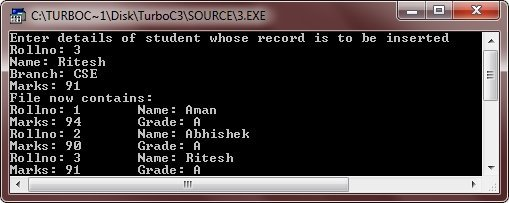## Deleting a Record in C++

To delete a record, following procedure is carried out :

(i) Firstly, determine the position of the record to be deleted, by performing a search in the file.

(ii) Keep copying the records other than the record to be delete in a temporary file say temp.dat.

(iii) Do not copy the record to be deleted to temporary file, temp.dat.

(iv) Copy rest of the records to temp.dat.

(v) Delete original file say marks.dat as :

`remove("marks.dat");`

(vi) Rename temp.dat as marks.dat as :

`rename("temp.dat", "marks.dat");`

For example program, just copy the above code, and make changes as told in the above steps to process the deletion of record.

## Modifying Data in C++

To modify a record, the file is opened in I/O mode and an important step is performed that gives the beginning address of record being modified. After the record is modified in memory, the file pointer is once again placed at the beginning position of this record and then record is rewritten. Following code illustrates it :

```class student
{
:
void modify();
}stud1;

fstream fio("marks.dat", ios::in | ios::out);
/* Read rollno whose data is to be modified */
long pos;
while(!fio.eof())
{
pos = fio.tellg()          // determine the beginning position of record
if(stud1.getrno() == rn)       // this is the record to be modified
{
stud1.modify();          // get the new data
fio.seekg(pos);       // place file pointer at the beginning record position
fio.write((char *) & stud1, sizeof(stud1));     // now write the modified record
}
}```

For example program, just copy the above code, and make changes, as shown in the above code fragment to process the modification of data in a file.

### More Examples

You can also go through the following examples, using files in C++:

Tools
Calculator